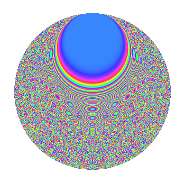# Properties

 Label 525.4.boLevel $525$ Weight $4$ Character orbit 525.bo Rep. character $\chi_{525}(4,\cdot)$ Character field $\Q(\zeta_{30})$ Dimension $960$ Sturm bound $320$

# Related objects

## Defining parameters

 Level: $$N$$ $$=$$ $$525 = 3 \cdot 5^{2} \cdot 7$$ Weight: $$k$$ $$=$$ $$4$$ Character orbit: $$[\chi]$$ $$=$$ 525.bo (of order $$30$$ and degree $$8$$) Character conductor: $$\operatorname{cond}(\chi)$$ $$=$$ $$175$$ Character field: $$\Q(\zeta_{30})$$ Sturm bound: $$320$$

## Dimensions

The following table gives the dimensions of various subspaces of $$M_{4}(525, [\chi])$$.

Total New Old
Modular forms 1952 960 992
Cusp forms 1888 960 928
Eisenstein series 64 0 64

## Trace form

 $$960q - 480q^{4} + 8q^{5} - 48q^{6} - 420q^{8} - 1080q^{9} + O(q^{10})$$ $$960q - 480q^{4} + 8q^{5} - 48q^{6} - 420q^{8} - 1080q^{9} - 18q^{10} - 84q^{11} + 132q^{14} + 84q^{15} + 1920q^{16} - 640q^{17} - 304q^{19} + 952q^{20} - 720q^{22} + 720q^{23} + 1152q^{24} - 210q^{25} + 360q^{28} - 96q^{29} + 576q^{30} + 330q^{31} + 800q^{34} + 204q^{35} - 8640q^{36} + 2490q^{38} + 438q^{40} - 576q^{41} + 1020q^{42} - 896q^{44} - 72q^{45} + 228q^{46} + 516q^{49} + 3932q^{50} - 4170q^{52} + 4320q^{53} - 216q^{54} - 2084q^{55} + 2700q^{56} - 3420q^{58} + 984q^{59} - 1614q^{60} + 2120q^{61} - 6960q^{62} + 180q^{63} + 11436q^{64} - 660q^{65} + 1056q^{66} - 4620q^{67} + 2208q^{69} + 7410q^{70} - 1472q^{71} + 1890q^{72} + 2280q^{73} + 2988q^{74} + 312q^{75} + 25544q^{76} + 1084q^{79} + 5944q^{80} + 9720q^{81} - 13840q^{83} + 6768q^{84} - 12088q^{85} + 336q^{86} - 6250q^{88} + 216q^{90} + 1884q^{91} + 13860q^{92} - 3104q^{94} + 4448q^{95} - 1182q^{96} + 13020q^{97} - 33720q^{98} + 1008q^{99} + O(q^{100})$$

## Decomposition of $$S_{4}^{\mathrm{new}}(525, [\chi])$$ into newform subspaces

The newforms in this space have not yet been added to the LMFDB.

## Decomposition of $$S_{4}^{\mathrm{old}}(525, [\chi])$$ into lower level spaces

$$S_{4}^{\mathrm{old}}(525, [\chi]) \cong$$ $$S_{4}^{\mathrm{new}}(175, [\chi])$$$$^{\oplus 2}$$

## Hecke characteristic polynomials

There are no characteristic polynomials of Hecke operators in the database